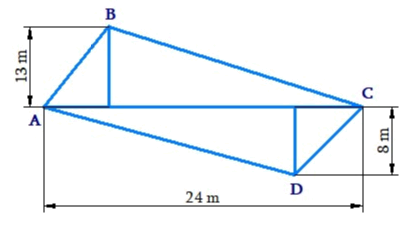# Ex.11.2 Q4 Mensuration Solution - NCERT Maths Class 8

Go back to  'Ex.11.2'

## Question

The diagonal of a quadrilateral shaped field is $$24\,\rm{ m}$$ and the perpendiculars dropped on it from the remaining opposite vertices are $$8\,\rm{ m}$$ and $$13\,\rm{ m.}$$ Find the area of the field.Video Solution
Mensuration
Ex 11.2 | Question 4

## Text Solution

What is Known?

The shape of the field is quadrilateral, and length of the diagonal and length of the perpendicular drop is given.

What is unknown?

Area of the field $$ABCD.$$

Reasoning:

A general quadrilateral can be split into two triangles by drawing one of its diagrams .

Area for quadrilateral will be the sum of area of two triangles.

Steps:

Area of quadrilateral $$ABCD$$

\begin{align} &= \! \text{Area of }\Delta \,\text{ABC} \! + \! \text{Area of }\Delta \,\text{ADC} \\ & = \! \frac{1}{2} \! \times \! (AC \! \times \! BE) \! + \! \frac{1}{2} \! \times \! (AC \! \times \! FD) \\ & = \! \frac{1}{2} \! \times \! AC(BE \! + \! FD) \\ & = \! \frac{1}{2} \! \times \! 24\,\text{m}(13\,\text{m} \! +8 \! \,\text{m}) \\& = \! \frac{1}{2} \! \times \! 24\,\text{m} \! \times \! 21\,\text{m} \\ & = \! 252\,{{\text{m}}^{2}}\end{align}

Thus, area of the field is $$252\,{{\rm{m}}^2}$$

Learn from the best math teachers and top your exams

• Live one on one classroom and doubt clearing
• Practice worksheets in and after class for conceptual clarity
• Personalized curriculum to keep up with school